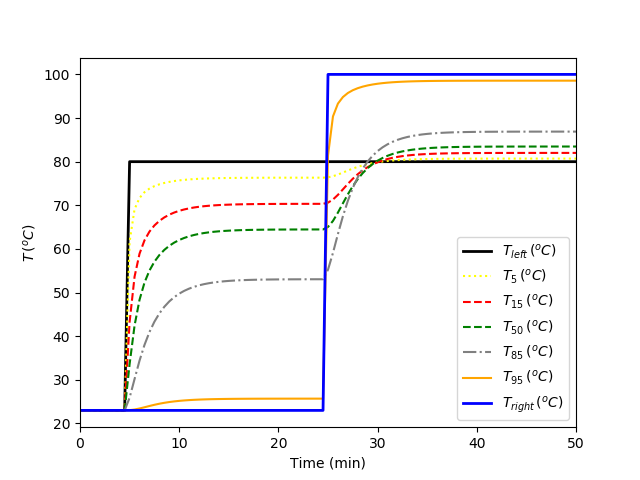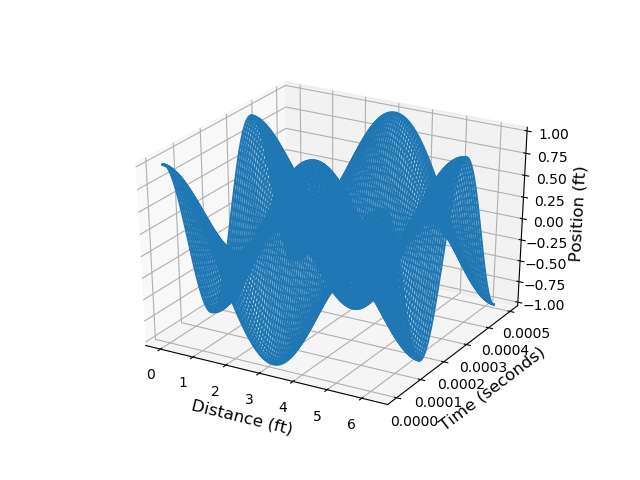### Partial Differential Equations in Python

When there is spatial and temporal dependence, the transient model is often a partial differntial equation (PDE). Orthogonal Collocation on Finite Elements is reviewed for time discretization. A similar approach can be taken for spatial discretization as well for numerical solution of PDEs. There are analytic (exact and closed form) solutions to PDEs but they are limited to ideal or simplified cases that may not be able to capture the full physics of the problem. For numerical solutions, Finite Element Analysis (FEA) is used to break the simulated object into a grid of discrete points. These discrete points are an approximation of the local quantity of interest. Boundary conditions are specified in space and time and are often the Manipulated Variables for the problem.

Once a PDEs is simulated, it can also be used in estimation or predictive control problems. There are examples of PDE models with Moving Horizon Estimation (MHE) and Model Predictive Control (MPC).

Two common PDEs are the heat equation and the wave equation. The wave equation describes the propagation of waves such as in water, sound, and seismic. The heat equation describes the transfer of heat as it flows from high temperature to low temperature regions.

#### Heat Equation

The heat equation in one dimension is a parabolic PDE. The one dimensional transient heat equation is contains a partial derivative with respect to time and a second partial derivative with respect to distance.

$$\rho c_p \frac{\partial T}{\partial t} = \frac{\partial}{\partial t} \left( k \frac{\partial T}{\partial t} \right) + \dot Q$$

with \rho as density, c_p as heat capacity, T as the temperature, k as the thermal conductivity, and \dot Q as the heat input rate. The heat equation is discretized in space to give a set of Ordinary Differential Equations (ODEs) in time.

Heated Rod (Left Boundary Condition)

The following simulation is for a heated rod (10 cm) with the left side temperature step to 100 oC. The heat transfers to the right by conduction and away from the rod by convection. The temperature at the tip is the lowest temperature because of heat lost by convection to the surrounding air.# The parabolic PDE equation describes the evolution of temperature
#  for the interior region of the rod. This model is modified to make
#  one end of the rod fixed and the other temperature at the end of the
#  rod calculated.
import numpy as np
from gekko import GEKKO
import matplotlib.pyplot as plt

# Steel rod temperature profile
# Diameter = 3 cm
# Length = 10 cm
seg      = 100              # number of segments
T_melt   = 1426             # melting temperature of H13 steel
pi       = 3.14159          # pi
d        = 3 / 100          # rod diameter (m)
L        = 10 / 100         # rod length (m)
L_seg    = L / seg          # length of a segment (m)
A        = pi * d**2 / 4    # rod cross-sectional area (m)
As       = pi * d * L_seg   # surface heat transfer area (m^2)
heff     = 5.8              # heat transfer coeff (W/(m^2*K))
keff     = 28.6             # thermal conductivity in H13 steel (W/m-K)
rho      = 7760             # density of H13 rod steel (kg/m^3)
cp       = 460              # heat capacity of H13 steel (J/kg-K)
Ts       = 23               # temperature of the surroundings (°C)
c2k      = 273.15           # Celcius to Kelvin

m = GEKKO()  # create GEKKO model

tf = 3000
nt = int(tf/30) + 1
m.time = np.linspace(0,tf,nt)
Th = m.MV(ub=T_melt)        # heater temperature (°C)
Th.value = np.ones(nt) * 23 # start at room temperature
Th.value[10:] = 100         # step at 300 sec

T = [m.Var(23) for i in range(seg)] # temperature of the segments (°C)

# Energy balance for the rod (segments)
# accumulation =
#    (heat gained from upper segment)
#  - (heat lost to lower segment)
#  - (heat lost to surroundings)
# Units check
# kg/m^3 * m^2 * m * J/kg-K * K/sec =
#     W/m-K   * m^2 *  K / m
#  -  W/m-K   * m^2 *  K / m
#  -  W/m^2-K * m^2 *  K

# first segment
m.Equation(rho*A*L_seg*cp*T.dt() == \
keff*A*(Th-T)/L_seg \
- keff*A*(T-T)/L_seg \
- heff*As*(T-Ts))
# middle segments
m.Equations([rho*A*L_seg*cp*T[i].dt() == \
keff*A*(T[i-1]-T[i])/L_seg \
- keff*A*(T[i]-T[i+1])/L_seg \
- heff*As*(T[i]-Ts) for i in range(1,seg-1)])
# last segment
m.Equation(rho*A*L_seg*cp*T[seg-1].dt() == \
keff*A*(T[seg-2]-T[seg-1])/L_seg \
- heff*(As+A)*(T[seg-1]-Ts))

# simulation
m.options.IMODE = 4
m.solve()

# plot results
plt.figure()
tm = m.time / 60.0
plt.plot(tm,Th.value,'r-',label=r'$T_{heater}\,(^oC)$')
plt.plot(tm,T.value,'k--',label=r'$T_5\,(^oC)$')
plt.plot(tm,T.value,'k:',label=r'$T_{15}\,(^oC)$')
plt.plot(tm,T.value,'k:',label=r'$T_{25}\,(^oC)$')
plt.plot(tm,T.value,'k-.',label=r'$T_{45}\,(^oC)$')
plt.plot(tm,T[-1].value,'b-',label=r'$T_{tip}\,(^oC)$')
plt.ylabel(r'$T\,(^oC$)')
plt.xlabel('Time (min)')
plt.xlim([0,50])
plt.legend(loc=4)
plt.show()

Heated Rod (Left and Right Boundary Conditions)

The following simulation is for the same heated rod (10 cm) as above but with the left and right side temperatures specified. The left is stepped up to 80 oC at about 5 minutes and the right is stepped up to 100 oC at about 25 minutes. The heat transfers to the center by conduction and away from the rod by convection.# The parabolic PDE equation describes the evolution of temperature
#  for the interior region of the rod. This model is modified to make
#  one end of the device fixed and the other temperature at the end of the
#  device calculated.
import numpy as np
from gekko import GEKKO
import matplotlib.pyplot as plt
import matplotlib.animation as animation

# Steel temperature profile
# Diameter = 3 cm
# Length = 10 cm
seg      = 100              # number of segments
T_melt   = 1426             # melting temperature of H13 steel
pi       = 3.14159          # pi
d        = 3 / 100          # diameter (m)
L        = 10 / 100         # length (m)
L_seg    = L / seg          # length of a segment (m)
A        = pi * d**2 / 4    # cross-sectional area (m)
As       = pi * d * L_seg   # surface heat transfer area (m^2)
heff     = 5.8              # heat transfer coeff (W/(m^2*K))
keff     = 28.6             # thermal conductivity in H13 steel (W/m-K)
rho      = 7760             # density of H13 steel (kg/m^3)
cp       = 460              # heat capacity of H13 steel (J/kg-K)
Ts       = 23               # temperature of the surroundings (°C)

m = GEKKO()  # create GEKKO model

tf = 3000
nt = int(tf/30) + 1
dist = np.linspace(0,L,seg+2)
m.time = np.linspace(0,tf,nt)
T1 = m.MV(ub=T_melt)        # temperature 1 (°C)
T1.value = np.ones(nt) * 23 # start at room temperature
T1.value[10:] = 80         # step at 300 sec

T = [m.Var(23) for i in range(seg)] # temperature of the segments (°C)

T2 = m.MV(ub=T_melt)        # temperature 2 (°C)
T2.value = np.ones(nt) * 23 # start at room temperature
T2.value[50:] = 100         # step at 300 sec

# Energy balance for the segments
# accumulation =
#    (heat gained from upper segment)
#  - (heat lost to lower segment)
#  - (heat lost to surroundings)
# Units check
# kg/m^3 * m^2 * m * J/kg-K * K/sec =
#     W/m-K   * m^2 *  K / m
#  -  W/m-K   * m^2 *  K / m
#  -  W/m^2-K * m^2 *  K

# first segment
m.Equation(rho*A*L_seg*cp*T.dt() == \
keff*A*(T1-T)/L_seg \
- keff*A*(T-T)/L_seg \
- heff*As*(T-Ts))
# middle segments
m.Equations([rho*A*L_seg*cp*T[i].dt() == \
keff*A*(T[i-1]-T[i])/L_seg \
- keff*A*(T[i]-T[i+1])/L_seg \
- heff*As*(T[i]-Ts) for i in range(1,seg-1)])
# last segment
m.Equation(rho*A*L_seg*cp*T[-1].dt() == \
keff*A*(T[-2]-T[-1])/L_seg \
- keff*A*(T[-1]-T2)/L_seg \
- heff*As*(T[-1]-Ts))

# simulation
m.options.IMODE = 4
m.solve()

# plot results
plt.figure()
tm = m.time / 60.0
plt.plot(tm,T1.value,'k-',linewidth=2,label=r'$T_{left}\,(^oC)$')
plt.plot(tm,T.value,':',color='yellow',label=r'$T_{5}\,(^oC)$')
plt.plot(tm,T.value,'--',color='red',label=r'$T_{15}\,(^oC)$')
plt.plot(tm,T.value,'--',color='green',label=r'$T_{50}\,(^oC)$')
plt.plot(tm,T.value,'-.',color='gray',label=r'$T_{85}\,(^oC)$')
plt.plot(tm,T.value,'-',color='orange',label=r'$T_{95}\,(^oC)$')
plt.plot(tm,T2.value,'b-',linewidth=2,label=r'$T_{right}\,(^oC)$')
plt.ylabel(r'$T\,(^oC$)')
plt.xlabel('Time (min)')
plt.xlim([0,50])
plt.legend(loc=4)
plt.savefig('heat.png')

# create animation as heat.mp4
fig = plt.figure(figsize=(5,4))
fig.set_dpi(300)

# store results in d
d = np.empty((seg+2,len(m.time)))
d = np.array(T1.value)
for i in range(seg):
d[i+1] = np.array(T[i].value)
d[-1] = np.array(T2.value)
d = d.T

k = 0
def animate(i):
global k
k = min(len(m.time)-1,k)
ax1.clear()
plt.plot(dist*100,d[k],color='red',label=r'Temperature ($^oC$)')
plt.text(1,100,'Elapsed time '+str(round(m.time[k]/60,2))+' min')
plt.grid(True)
plt.ylim([20,110])
plt.xlim([0,L*100])
plt.ylabel(r'T ($^oC$)')
plt.xlabel(r'Distance (cm)')
plt.legend(loc=1)
k += 1

anim = animation.FuncAnimation(fig,animate,frames=len(m.time),interval=20)
# requires ffmpeg to save mp4 file
#  available from https://ffmpeg.zeranoe.com/builds/
#  add ffmpeg.exe to path such as C:\ffmpeg\bin\ in
#  environment variables
try:
anim.save('heat.mp4',fps=10)
except:
print('requires ffmpeg to save mp4 file')
plt.show()

#### Wave Equation

The wave equation solution is described in the GEKKO Paper and work on Artificial Lift Rod Pumping and in the journal publication Model Predictive Automatic Control of Sucker Rod Pump System with Simulation Case Study (2019).import numpy as np
import matplotlib.pyplot as plt
from gekko import*
from mpl_toolkits.mplot3d.axes3d import Axes3D

tf = .0005
npt = 100
xf = 2*np.pi
npx = 100
time = np.linspace(0,tf,npt)
xpos = np.linspace(0,xf,npx)

m = GEKKO()
m.time = time

def phi(x):
phi = np.cos(x)
return phi

def psi(x):
psi = np.sin(2*x)
return psi

x0 = phi(xpos)
v0 = psi(xpos)
dx = xpos-xpos
a = 18996.06
c = m.Const(value = a)
dx = m.Const(value = dx)
u = [m.Var(value = x0[i]) for i in range(npx)]
v = [m.Var(value = v0[i]) for i in range(npx)]
[m.Equation(u[i].dt()==v[i]) for i in range(npx)]
m.Equation(v.dt()==c**2 * \
(u - 2.0*u + u[npx-1])/dx**2 )
[m.Equation(v[i+1].dt()== \
c**2 * (u[i+2] - 2.0*u[i+1] + u[i])/dx**2) \
for i in range(npx-2) ]
m.Equation(v[npx-1].dt()== c**2 * \
(u[npx-2] - 2.0*u[npx-1] + u)/dx**2 )
m.options.imode = 4
m.options.solver = 1
m.options.nodes = 3

m.solve()

# re-arrange results for plotting
for i in range(npx):
if i ==0:
ustor = np.array([u[i]])
tstor = np.array([m.time])
else:
ustor = np.vstack([ustor,u[i]])
tstor = np.vstack([tstor,m.time])
for i in range(npt):
if i == 0:
xstor = xpos
else:
xstor = np.vstack([xstor,xpos])
xstor = xstor.T
t = tstor
ustor = np.array(ustor)

fig = plt.figure()
ax.set_xlabel('Distance (ft)', fontsize = 12)
ax.set_ylabel('Time (seconds)', fontsize = 12)
ax.set_zlabel('Position (ft)', fontsize = 12)
ax.set_zlim((-1,1))
p = ax.plot_wireframe(xstor,tstor,ustor,\
rstride=1,cstride=1)
fig.savefig('wave_3d.png', Transparent=True)

plt.figure()
plt.contour(xstor, tstor, ustor, 150)
plt.colorbar()
plt.xlabel('X')
plt.ylabel('Time')
plt.savefig('wave_contour.png', Transparent=True)
plt.show()